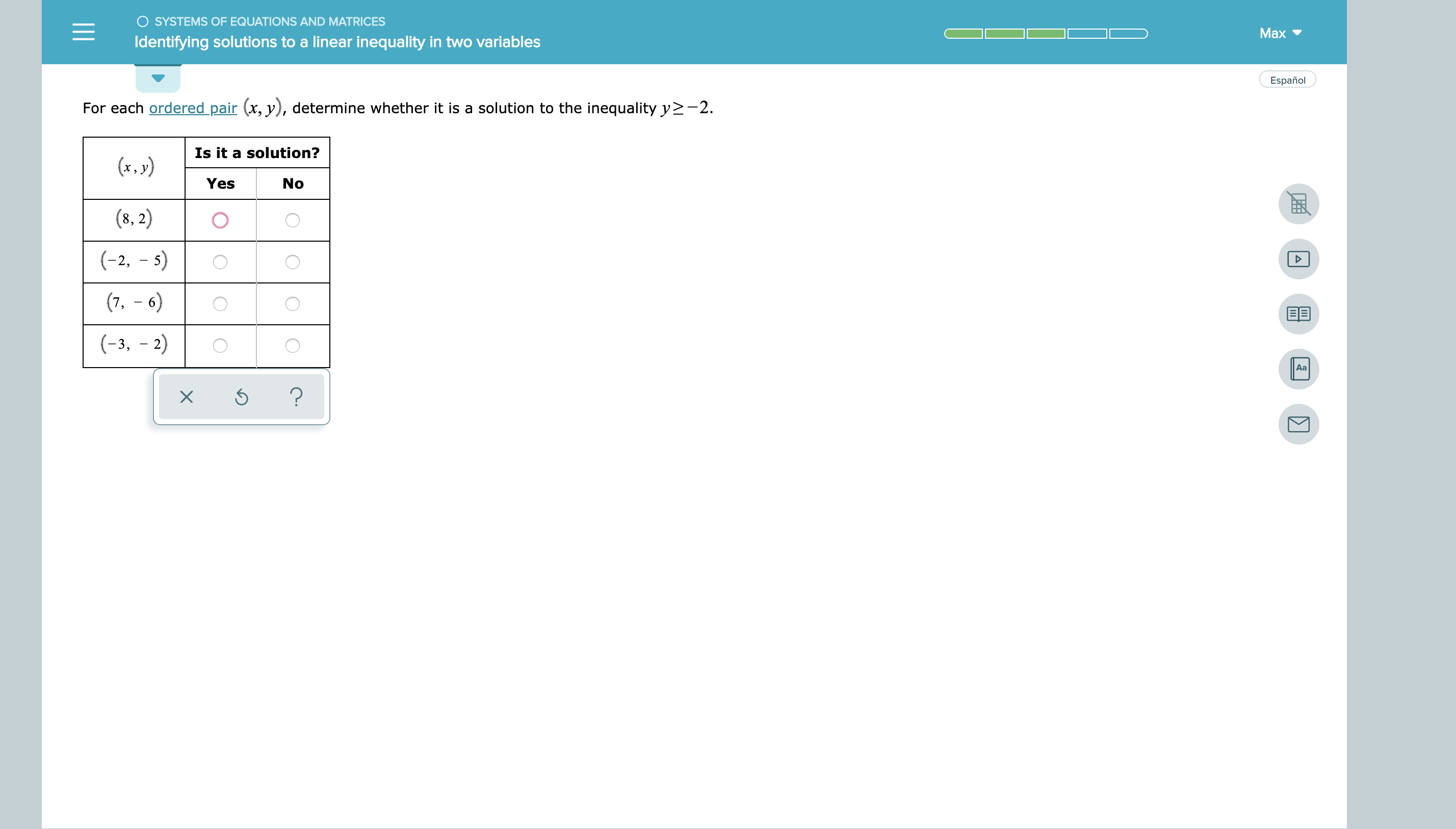OSYSTEMS OF EQUATIONS AND MATRICESMaxIdentifying solutions to a linear inequality in two variablesEspañolFor each ordered pair (x, y), determine whether it is a solution to the inequality y-2Is it a solution?(x,y)YesNo(8,2)(-2, 5)(7,- 6)BE(-3, -2)Aa?X

Question

see attachmenthelp_outlineImage TranscriptioncloseOSYSTEMS OF EQUATIONS AND MATRICES Max Identifying solutions to a linear inequality in two variables Español For each ordered pair (x, y), determine whether it is a solution to the inequality y-2 Is it a solution? (x,y) Yes No (8,2) (-2, 5) (7,- 6) BE (-3, -2) Aa ? X fullscreen
Step 1

To satisfy y>= -2 the coordinates must ha...

Want to see the full answer?

See Solution

Want to see this answer and more?

Our solutions are written by experts, many with advanced degrees, and available 24/7

See Solution
Tagged in

Other